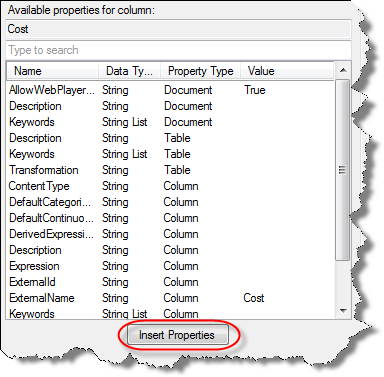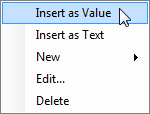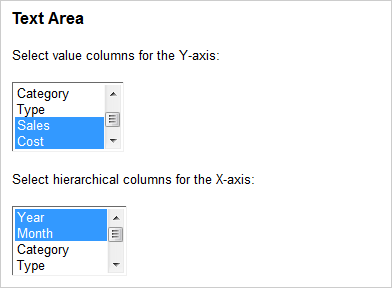# Properties in Expressions

There are three types of properties available in TIBCO Spotfire: document properties, data table properties and column properties. All of these can be used in expressions that control one or many settings. The properties can be interpreted in two different ways, either as actual values or as text values that can be used instead of column names or measures.

Insert as Text

When you want to use a string property in an expression where it should be interpreted as a column name or a measure you must use a specific syntax. The name of the property should be enclosed in the following: “\${“ and “}”. So, for a document property this could look something like: \${MyProperty}. For a data table property, the first part should be the data table name within the symbols specified above, followed by a period and the property name also within curly brackets: \${My Data Table}.{MyProperty}. For a column property, the column name is also required: \${My Data Table}.{Column Name}.{MyProperty}.

 Property call in expression Description \${MyProperty} Denotes the document property named MyProperty. \${My Data Table}.{MyProperty} Denotes the data table property named MyProperty defined for the data table "My Data Table". \${My Data Table}.{Column Name}.{MyProperty} Denotes the column property named MyProperty defined for the column "Column Name" in the data table "My Data Table". \$map("template", "concatenation string") The \$map() function is used to map list-valued properties to a single string. The first argument is a template to use for each value in the list and the second argument is a specification of how the list values should be connected in the resulting expression. See List-Valued Properties (Multiple Select) below for more information. Examples: \$map("sum([\${PropertyName}])", ",") <\$map("[\${PropertyName}]", " NEST")> \$esc(expression) Replaces "]" in column names with "]]" and encloses the escaped column names in "[" and "]". The argument is an expression which could be a property value or a property function that starts with a dollar sign (\$). Examples: \$esc(\${MyProperty}) \$esc(\$csearch([Data Table], "Col*")) \$csearch([Data Table], "search string") The \$csearch() function is used to select a number of columns from a data table using a limiting search expression. The first argument is a data table and the second argument is a string that contains the search expression determining which column names should be returned. The function returns a list of the (unescaped) column names from the data table that match the search expression. Examples: \$csearch([Data Table],"*") \$csearch([Data Table], "Col*")

When the \$ sign is encountered in an expression, the property will be replaced by its corresponding value before the actual expression is evaluated (a preprocessing step). This means that if you type an expression like: sum(\${Property1}) -\${Property1}-\${Property2}, and the value of Property1 is the column name Column1, and the value of Property2 is Column2, then the resulting expression will be: sum([Column1]) -[Column1]-[Column2].

If you do not want the property value to be interpreted as a column name but as a literal string value, you should put the property call within quotation marks. For example, if you have a property called "MyProperty" with the value "MyValue", the expression \${MyProperty} would return the resulting expression MyValue, where a column called "MyValue" is retrieved. However, the expression "\${MyProperty}" would return the text string "MyValue" instead. If the string value consists of several words (e.g., "My Value"), then you should use \$esc() or put brackets around the property expression in order to return a column: [\${MyProperty}]. See General Syntax for more information about column names.

Note that it is very important to use the correct syntax for the expression at all times. Otherwise you may end up with a different result than you expected. If a property is inserted as a column where the expression expected a string, the first value of the specified column will be retrieved. For string columns, the result may be a valid expression, but for a column of another data type, you may receive an error.

If a property is inserted using the Insert Properties button in the Add calculated column dialog or in the Custom Expression dialog, the property will automatically be added using the text (preprocessor) syntax.You can also right-click the property in the Available properties for column field and select Insert as Text from the pop-up menu.

If a property containing a column name is to be used on an axis, there is a shortcut called Set from Property available from the pop-up menu on the column selectors. If any changes to the automatically added syntax are required, you must use the Custom Expression dialog to modify the expression. Right-click the column selector and select Remove All if you want to remove the property expression from the axis.

A property can also be used to specify an aggregation measure. For example, you can define a property called MyMeasure with the default value "sum". If such a property is to be used in an expression you need to manually add parentheses and arguments for the measure in the expression where it is used. You can then create a property selector in a text area displaying a list of fixed aggregation measures that you want to be available in an axis expression and let web client users change the axis expression using the property selector.

Since string properties inserted as text in most cases will be interpreted as column names, you may encounter situations where you do not achieve the expected result when creating a custom expression. If the string property is to be interpreted as a value (e.g., in conditional expressions) it needs to be surrounded by quotation marks. For example, if you want to replace the string "ProductA" used in an axis expression condition such as Sum(if(([Product])=("ProductA"),[Quantity],null)) with a document property, then the document property needs to be surrounded by quotation marks in order to make the expression work:

Sum(if ( [Product] = "\${MyProduct}",[Quantity],null))

You can also insert the property as a function rather than as text in order to retrieve the same results (see also Insert as Value below):

Sum(if ( [Product] = DocumentProperty("MyProduct"),[Quantity],null))

Insert as Value

When the value of a property is to be part of an expression, the recommendation is to use the standard property functions: ColumnProperty(), DataTableProperty() and DocumentProperty() to encapsulate the property name. For example, use this syntax to write an expression with a document property holding an exchange rate times a value column expressed in some currency to be converted:

DocumentProperty("ExchangeRate")*[Value Column]

Note that the property name should always be written within quotation marks when property functions are applied. If you want a property to be interpreted as a value, you should right-click on the property in the Available properties for column field and select Insert as Value from the pop-up menu.You can of course also manually edit the syntax for the property.

List-Valued Properties (Multiple Select)

Multiple-select properties, or list-valued properties, are based on a list of values instead of a single value. They can be created when defining a list box (multiple select) property control. A list-valued property cannot be used in all places where a regular single-valued property can be applied, but it is an effective way to specify multiple columns on an axis.List-valued properties often require some additional manual work when used in expressions. However, you will in most cases get a hint of what the expression should look like in the current context when inserting the property using the Insert Properties button.

When a list-valued property is added as text to a custom expression (for example, on a continuous axis like the Y-axis in a Line Chart or the value axis in a Bar Chart) the syntax will be something like \$map("sum([\${PropertyName}])", ","). In this example, the default aggregation is set to "sum", so if you want to use a different aggregation you need to manually edit the expression. The concatenation string is set to a comma, which means that each list value should be interpreted as a separate column. If the PropertyName property contains a list with three columns: "ColumnA", "ColumnB" and "ColumnC", the resulting expression after expansion is sum([ColumnA]),sum([ColumnB]),sum([ColumnC]). If the concatenation string is changed to a "+", then the axis would show the sum of the values from all columns included in the list: sum([ColumnA])+sum([ColumnB])+sum([ColumnC]).

For a categorical axis, such as the X-axis in a Line Chart or the category axis in a Bar Chart, you want to display a hierarchy rather than some calculated values. The map expression must then use angle brackets, "<>", and either NEST or CROSS to define what combinations to show. For example:

<\$map("[\${PropertyName}]", " NEST")>

Note: As always when setting up expressions with multiple columns, you need to make sure that the columns you use are of the same type and that they match the rest of the expression. For example, you cannot mix categorical and continuous columns on some axes, nor can you use categorical columns in an expression using any type of aggregation.

More Expression Examples

If nothing else is stated, the expression examples below assume that you have a data table called Data Table containing three integer columns called "Column 1", "Column 2", and "Sales":

Column 1, Column 2, Sales

1,2,3

4,5,6

 Requested result Expression example Resulting expression Sum all integer columns in the data table called Data Table. \$map("sum([\$csearch([Data Table],"datatype:int")])","+") sum([Column 1])+sum([Column 2])+sum([Sales]) Return a list of all columns in the data table called Data Table. \$csearch([Data Table],"*") Column1,Column 2,Sales Return an escaped column name from a property (MyProperty) with the value "Column name with bracket (])". \$esc(\${MyProperty}) [Column name with bracket (]])] Use a list-valued property expression as input to a data function. In the example, the property MyListProperty contains three column names: Column 1, Column 2, and Sales. \$map("[Data Table].[\${MyListProperty}]", ",") [Data Table].[Column 1],[Data Table].[Column 2],[Data Table].[Sales] Use multiple columns on an axis where one of the columns is retrieved via a property control. In the examples, the property MyProperty has the value Column 2. [Column 1],[\${MyProperty}] or <[Column 1] NEST [\${MyProperty}]> or Sum([Column 1]), Sum(\${MyProperty}]) etc. [Column 1],[Column 2] or <[Column 1] NEST [Sales]> or Sum([Column 1]), Sum(Column 2]) etc. Change the display name of multiple columns on an axis using a list-valued property. In the example, the property MyListProperty contains three column names: Column 1, Column 2 and Sales. \$map("Sum([\${MyListProperty}]) as [\${MyListProperty}]", ",")       All list-valued properties in the expression must be of the same size. Sum([Column 1]) as [Column 1],Sum([Column 2]) as [Column 2],Sum([Sales]) as [Sales]